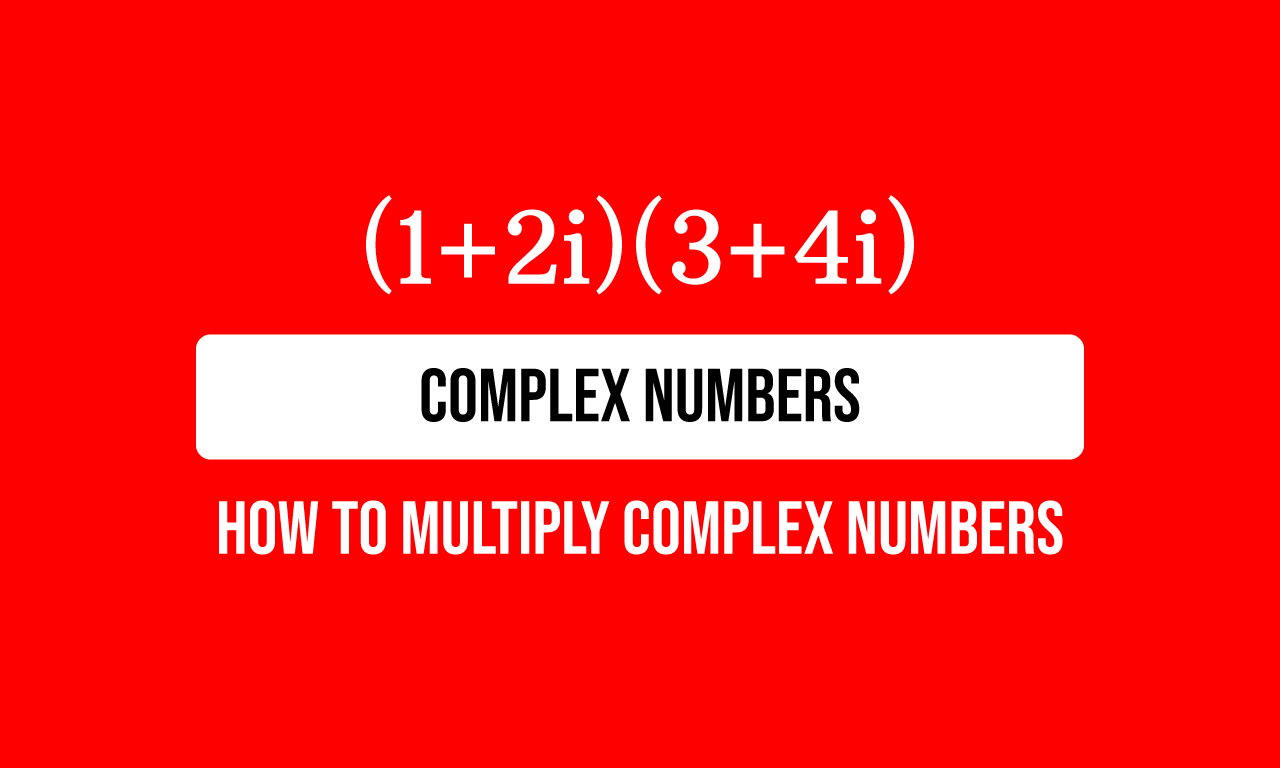# Evaluate $(1+2i)(3+4i)$

The product of one plus two times imaginary unit and three plus four times imaginary unit is the given mathematical expression in this math problem.$(1+2i)(3+4i)$

The product of $1$ plus $2i$ and $3$ plus $4i$ is a mathematical representation for the multiplication of them. Therefore, the above mathematical expression can be written as follows.

$=\,\,\,$ $(1+2i) \times (3+4i)$

### Trick to avoid confusion in multiplication

Multiplying the terms in the complex number $3$ plus $4i$ by another complex number $1$ plus $2i$ confuses some learners. So, let’s denote the complex number $1$ plus $2i$ by a variable. In this problem, the complex number $1+2i$ is denoted by a variable $z$.

$=\,\,\,$ $z \times (3+4i)$

### Multiply the terms by their coefficient

The complex $3+4i$ is a binomial and its terms are multiplied by a variable $z$. So, the terms $3$ and $4i$ can be multiplied by the variable $z$ as per the distributive property of multiplication over the addition.

$=\,\,\,$ $z \times 3$ $+$ $z \times 4i$

The use of variable $z$ is over. So, replace the variable $z$ by its actual value in the above mathematical expression.

$=\,\,\,$ $(1+2i) \times 3$ $+$ $(1+2i) \times 4i$

According to the commutative property of multiplication, the positions of the factors can be changed in each term.

$=\,\,\,$ $3 \times (1+2i)$ $+$ $4i \times (1+2i)$

Now, use the distributive property one more time in each term to distribute the coefficient over the addition of the terms.

$=\,\,\,$ $3 \times 1$ $+$ $3 \times 2i$ $+$ $4i \times 1$ $+$ $4i \times 2i$

### Simplify the Mathematical expression

The complex number $1$ plus $2i$ is multiplied by another complex number $3$ plus $4i$. The multiplication of them formed a mathematical expression. Now, it is time to simplify the mathematical expression to find the product of the given complex numbers $1$ plus $2i$ and $3$ plus $4i$.

$=\,\,\,$ $3$ $+$ $6i$ $+$ $4i$ $+$ $8i^2$

Second and third terms are like terms in the above mathematical expression. Therefore, add the like terms $6i$ and $4i$ to find the sum of them.

$=\,\,\,$ $3$ $+$ $10i$ $+$ $8i^2$

$=\,\,\,$ $3$ $+$ $10i$ $+$ $8 \times i^2$

According to the complex numbers, the square of imaginary unit is negative one.

$=\,\,\,$ $3$ $+$ $10i$ $+$ $8 \times (-1)$

$=\,\,\,$ $3$ $+$ $10i$ $-$ $8$

Now, use the commutative property to write the terms in an order for our convenience.

$=\,\,\,$ $3$ $-$ $8$ $+$ $10i$

Look at the first and second terms. They are numbers. So, subtract the number $8$ from number $3$ to find difference of them.

$=\,\,\,$ $-5+10i$

Latest Math Topics
Jun 26, 2023
Jun 23, 2023

Latest Math Problems
Jul 01, 2023
Jun 25, 2023
###### Math Questions

The math problems with solutions to learn how to solve a problem.

Learn solutions

Practice now

###### Math Videos

The math videos tutorials with visual graphics to learn every concept.

Watch now

###### Subscribe us

Get the latest math updates from the Math Doubts by subscribing us.